# Direct Proportion

Here we will learn about direct proportion, including what direct proportion is and how to solve direct proportion problems. We will also look at solving word problems involving direct proportion.

There are also direct proportion worksheets based on Edexcel, AQA and OCR exam questions, along with further guidance on where to go next if you’re still stuck.

## What is direct proportion?

Direct proportion is a type of proportionality relationship. For direct proportion, as one value increases, so does the other value and conversely, as one value decreases, so does the other value.

The symbol \textbf{∝} represents a proportional relationship.

If y is directly proportional to x, we can write this relationship as:

\bf{y\propto{x}}

Direct proportion is useful in numerous real life situations such as exchange rates, conversion between units, and fuel prices.

### What is direct proportion?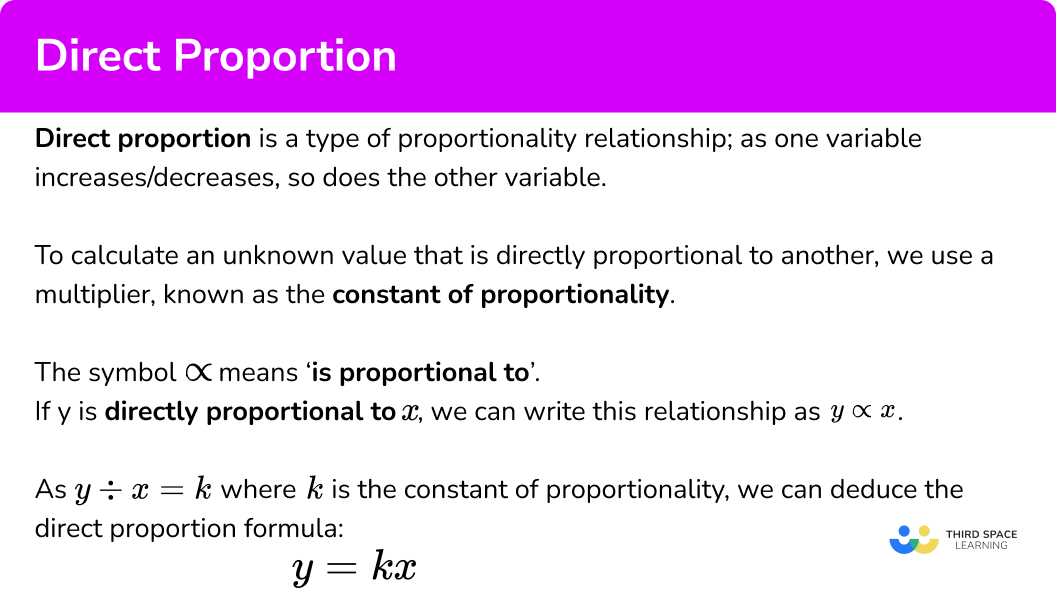### Direct proportion formula

The direct proportion formula allows us to express the relationship between two variables, using an equivalence relationship; the formula contains an equals symbol (=) instead of the proportionality symbol (\propto).

When y is directly proportional to x, the value of y \div x is a constant value. This is known as the constant of proportionality and we use the letter k to denote this number.

Given that k=y\div{x}, we can rearrange this formula to make y the subject, and hence obtain the standard format of the direct proportion formula:

y=kx

Step-by-step guide: Direct proportion formula

### Graphs representing a direct proportion between two variables

Proportional relationships can also be represented graphically.

If we sketched the straight line graph for the equation y=kx, the line must go through the origin (0,0) as the value of the y -intercept is 0, and the gradient of the line is equal to the value of k.

Step-by-step guide: y=mx+c.

Note, the value of y can be proportional to other powers of x including x^{2}, x^{3}, or even \sqrt{x}. Each of these has a different algebraic and graphical representation.

Step-by-step guide: Directly proportional graphs / inversely proportional graphs

## How to use direct proportion

In order to work out an unknown value given a directly proportional relationship:

1. Write down the direct proportion formula.
2. Determine the value of \textbf{k} .
3. Substitute \textbf{k} and the known value into the direct proportion formula.
4. Solve the equation.

### Explain how to use direct proportion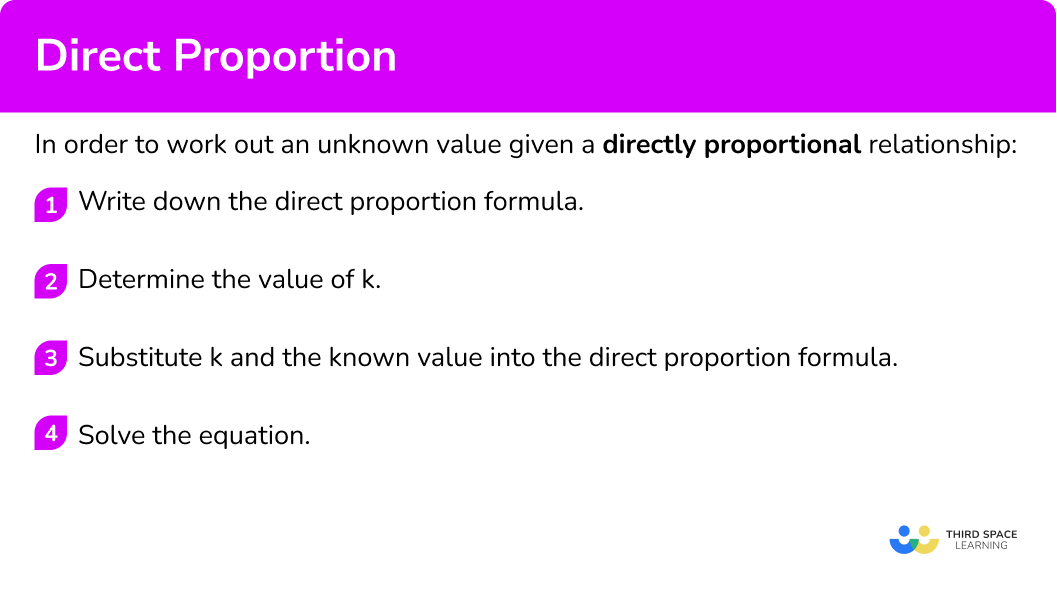## Direct proportion examples

### Example 1: y is directly proportional to x (table)

Given that y is directly proportional to x, calculate the value for y when x=6.

1. Write down the direct proportion formula.

As y \propto x we can state the formula y=kx.

2Determine the value of \textbf{k} .

From the table, we can see that when x=2, y=5. By substituting these values into the formula, we get the value of k.

\begin{aligned} 5&=k\times{2}\\\\ k&=5\div{2}\\\\ k&=2.5 \end{aligned}

3Substitute \textbf{k} and the known value into the direct proportion formula.

Substituting k=2.5 into the formula, we have:

y=2.5x

To find the value for y when x=6, substituting x=6 into the equation, we have

y=2.5\times{6}

4Solve the equation.

\begin{aligned} y&=2.5\times{6}\\\\ y&=15 \end{aligned}

### Example 2: y is directly proportional to x (table)

Let y\propto{x}. Calculate the value for x when y=32.

Write down the direct proportion formula.

Determine the value of \textbf{k} .

Substitute \textbf{k} and the known value into the direct proportion formula.

Solve the equation.

### Example 3: y is directly proportional to x2 (table)

Let y be directly proportional to x^2. Calculate the missing value p.

Write down the direct proportion formula.

Determine the value of \textbf{k} .

Substitute \textbf{k} and the known value into the direct proportion formula.

Solve the equation.

### Example 4: y is directly proportional to x (worded problem)

A florist uses ribbon to trim bunches of flowers.

80 \ cm of ribbon is needed to make the trimmings on 2 bunches of flowers.

The florist has 9 bunches of flowers to trim today.

How much ribbon will be needed?

Write down the direct proportion formula.

Determine the value of \textbf{k} .

Substitute \textbf{k} and the known value into the direct proportion formula.

Solve the equation.

### Example 5: y is directly proportional to x2 (worded problem)

The surface area A of a cube is directly proportional to the square of the side length L. A cube with side length 3cm has a surface area of 54cm^{2}.

Determine the side length of a cube with a surface area of 600cm^{2}.

Write down the direct proportion formula.

Determine the value of \textbf{k} .

Substitute \textbf{k} and the known value into the direct proportion formula.

Solve the equation.

### Example 6: y is directly proportional to the cube root of x (worded problem)

Let D represent the density of an object, and V=x^3 represent the volume of the object in terms of the side length x. A block of ice has a density of 0.9g/cm^3 and a volume of 10.8cm^{3}.

Given that D \propto V, determine the side length of a block of ice with the same mass, but a density of 0.92g/cm^{3}. Write your answer to 2 decimal places.

Write down the direct proportion formula.

Determine the value of \textbf{k} .

Substitute \textbf{k} and the known value into the direct proportion formula.

Solve the equation.

### Common misconceptions

• Direct proportion can be non-linear

If we represented the direct proportion formula y=kx using a graph, the line would be straight, going through the origin with gradient k. There are many nonlinear direct proportion relationships such as y=kx^2 (a quadratic graph), y=kx^3 (a cubic graph), or y= \sqrt{x} (a radical graph).

These are nonlinear functions as each graph is not a straight line, but x and y are still directly proportional to one another.

• The \textbf{y} -intercept is not equal to \bf{0}

Take the general equation of a straight line y=mx+c. The values of x and y are directly proportional if and only if c=0 as the gradient m describes the rate of change between the two variables ( m could be described as the constant of proportionality here). If c 0, the two variables are not directly proportional.

• Summative relationship rather than a multiplicative relationship

When using a table, the next value along for x is 3 more than the current value. The value for y is calculated by adding 3 to this value. This uses a summative relationship, rather than the required multiplicative relationship between two variables (the multiplier being the constant of proportionality, k ).

• Mixing up direct and inverse proportion

For direct proportion, the constant of proportionality k is the ratio of the two variables such as k=y \div x. For inverse proportion, k is the product of the two variables, such as k=xy.

Step-by-step guide: Inverse proportion (coming soon)

• Take care with writing money

Money is used in many direct proportion word problems. If an answer is 5.3 you may be tempted to write it as £5.3, but the correct way of writing it would be £5.30.

## Related lessons on direct proportion

Direct proportion is part of our series of lessons to support revision on proportion. You may find it helpful to start with the main proportion lesson for a summary of what to expect, or use the step by step guides below for further detail on individual topics. Other lessons in this series include:

### Practice direct proportion questions

1. y is directly proportional to x. Find the missing value.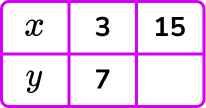10354530y\propto{x} and so y=kx. At (3,7),

\begin{aligned} 7&=k\times{3}\\\\ k&=7\div{3}=\frac{7}{3} \end{aligned}

So y=\frac{7}{3}x

When x=15, \ y=\frac{7}{3}\times{15}=7\times{5}=35

2. y is directly proportional to x. Find the missing value.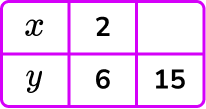2.51135y\propto{x} and so y=kx. At (2,6),

\begin{aligned} 6&=k\times{2}\\\\ k&=6\div{2}=3 \end{aligned}

So y=3x

When y=15,

\begin{aligned} 15&=3\times{x}\\\\ 15\div{3}&=x\\\\ x&=5 \end{aligned}

3. Given that y \propto x^2 , calculate the value of w.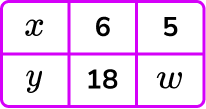151712.53y\propto x^2 and so y=kx^{2}. At (6,18),

\begin{aligned} 18&=k\times{6}^{2}\\\\ 18&=k\times{36}\\\\ 18\div{36}&=k\\\\ k&=0.5 \end{aligned}

So y=0.5x^{2}

When x=5,

\begin{aligned} y&=0.5\times{5}^{2} \\\\ y&=0.5\times{25} \\\\ y&=12.5 \end{aligned}

4. 3 pens cost £2.07. Find the cost of 13 pens.

£26.91£12.07£8.97£22.77As the cost c is directly proportional to the number of pens p, we can state c\propto{p}. This means that c=kp where k is the constant of proportionality. As c=2.07 when p=3,

\begin{aligned} 2.07&=k\times{3} \\\\ 2.07\div{3}&=k \\\\ k&=0.69 \end{aligned}

Each pen costs £0.69.

Now p=13, so

\begin{aligned} c&=0.69\times{13}\\\\ c&=8.97 \end{aligned}

13 pens cost £8.97.

5. The area of a circle is directly proportional to the square of the radius. Circle X has an area of 113.097cm^2 and a radius of 6cm. Circle Y has a radius of 4cm. Calculate the area of circle Y.

75.4cm^2 \ (1dp)18.8cm^2 \ (1dp)254.5cm^2 \ (1dp)50.3cm^2 \ (1dp)Let A represent the area of a circle, and r represent the radius of the circle, then A \propto r^2 so A=kr^{2}.

When A=113.097, \ r=6 and so

\begin{aligned} 113.097&=k\times{6}^{2}\\\\ 113.097&=k\times{36}\\\\ 113.097\div{36}&=k\\\\ k&=3.14158\dot{3} \end{aligned}

Now, A=3.14158\dot{3} \times r^2 and we know for circle Y, \ r=4.

Substituting this into the equation, we have:

\begin{aligned} A&=3.14158\dot{3}\times{4}^{2}\\\\ &=3.14158\dot{3}\times{16}\\\\ &=50.265\dot{3}\\\\ &=50.3\text{cm}^{2}\text{ (1dp)} \end{aligned}

6. The number of hours of sleep, h, is directly proportional to the square root of the number of marks achieved in an exam, m. The total marks in the exam is 100.

Identical twins Mark and Guy each sit the same exam. Mark had 6.5 hours of sleep and scored 64 marks in the exam. How many hours of sleep did Guy have to achieve 81 marks in the exam?

7.3125 hours12.462 hours (3dp)8.227 hours (3dp)5.\bar{7} hoursThe proportional relationship between the number of hours and the number of marks in the exam is written as h\propto\sqrt{m} and so h=k\sqrt{m}.

When h=6.5, \ m=64 and so

\begin{aligned} 6.5&=k\times\sqrt{64}\\\\ 6.5&=k\times{8}\\\\ 6.5\div{8}&=k\\\\ k&=\frac{13}{16} \end{aligned}

Now, h=\frac{13}{16}\sqrt{m}. As Guy scored 81 marks in the exam, m=81 and so we can substitute this into the equation to get:

\begin{aligned} h&=\frac{13}{16}\sqrt{81}\\\\ &=\frac{13}{16}\times{9}\\\\ &=\frac{117}{16}=7.3125 \end{aligned}

Guy had 7.3125 hours of sleep.

### Direct proportion GCSE questions

1. Crisps cost £3 for 12 packets.

Find the cost of 20 packets of crisps.

(3 marks)

k=3 \div 12=0.25

(1)

C=0.25 \times 20

(1)

£5.00

(1)

2. A force, F, of 128 Newtons, is applied to an area, A, of 2.8m^{2}.

Given that F \propto A, calculate the force required to apply the same amount of pressure over an area of 1.6m^{2}.

(3 marks)

k=128\div{2.8}=\frac{320}{7}=45.71428571…

(1)

F=\frac{320}{7}\times{1.6}

(1)

F=73.14N\text{ (2dp)}

(1)

3. Here are the ingredients needed to make 15 brownies

50 \ g chocolate

125 \ g margarine

225 \ g sugar

2 eggs

50 \ g plain flour

1 teaspoon baking powder

Orla wants to make 36 brownies.

She only has 320 \ g of margarine and 500 \ g of sugar.

She has plenty of the other ingredients

Does she have enough?

(4 marks)

125\div 15\times 36=300

(1)

Orla has enough margarine as 320 \ g is more than 300 \ g

(1)

225\div 15\times 36=540

(1)

Orla does NOT have enough sugar as 500 \ g is less than 540 \ g

(1)

4. A windup toy car is being held stationary on a table, ready to move. The displacement S is directly proportional to the square of the time T the object is moving.

(a) Using the information in the table, determine the displacement of the windup toy after 10 seconds.

State the direct proportion equation used in your solution.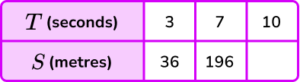(b) Hence determine how many seconds the windup toy has moved when he has travelled 144m.

(7 marks)

(a)

k=\frac{S}{T^{2}}=\frac{36}{3^{2}}=4\textbf{ or }k=\frac{S}{T^{2}}=\frac{196}{7^{2}}=4

(1)

S=4T^{2}

(1)

S=4\times{10}^{2}

(1)

S=400m

(1)

(b)

144=4T^{2}

(1)

36=T^2

(1)

T=6\text{seconds}

(1)

## Learning checklist

You have now learned how to:

• Solve problems involving direct proportion

## Still stuck?

Prepare your KS4 students for maths GCSEs success with Third Space Learning. Weekly online one to one GCSE maths revision lessons delivered by expert maths tutors.

Find out more about our GCSE maths tuition programme.